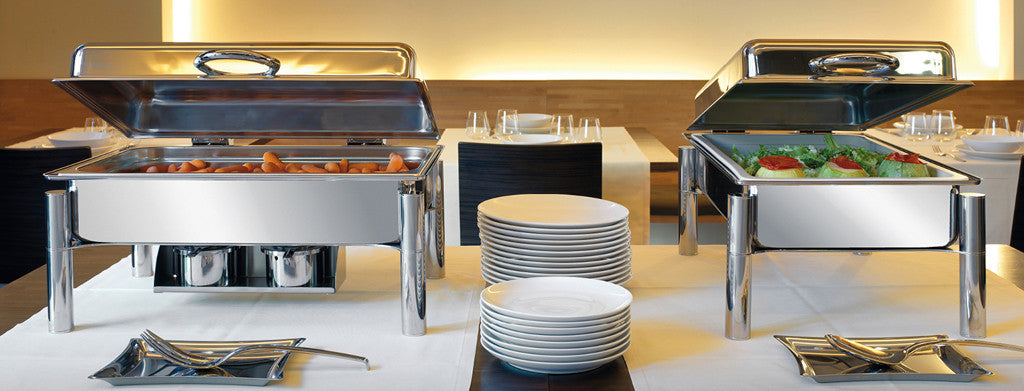Shopping Cart

### Refine

View all

#### Price# Buffet Presentation£16.99 each (ex VAT)£35.99 each (ex VAT)£67.99 each (ex VAT)£59.25 each (ex VAT) £79.00 Regular price£21.75 each (ex VAT) £29.00 Regular price£15.75 each (ex VAT) £21.00 Regular price£31.50 each (ex VAT) £42.00 Regular price£21.00 each (ex VAT)£15.00 each (ex VAT)£18.00 each (ex VAT)£13.00 each (ex VAT)£11.00 each (ex VAT)£10.00 each (ex VAT)£9.00 each (ex VAT)£16.00 each (ex VAT)£13.00 each (ex VAT)£360.67 each (ex VAT)£549.17 each (ex VAT)£627.25 each (ex VAT)£815.75 each (ex VAT)£1,195.59 each (ex VAT)£888.99 each (ex VAT)£1,030.25 each (ex VAT)£1,287.89 each (ex VAT)£1,013.43 each (ex VAT)£1,122.56 each (ex VAT)£1,303.76 each (ex VAT)£1,020.41 each (ex VAT)£1,286.53 each (ex VAT)£716.55 each (ex VAT)£886.95 each (ex VAT)£1,080.44 each (ex VAT)£743.73 each (ex VAT)£591.75 each (ex VAT)£580.48 each (ex VAT)£1,396.07 each (ex VAT)£1,144.85 each (ex VAT)£1,378.84 each (ex VAT)£1,172.75 each (ex VAT)£840.99 each (ex VAT)£979.25 each (ex VAT)£750.16 each (ex VAT)£660.45 each (ex VAT)£715.53 each (ex VAT)£656.77 each (ex VAT)£399.00 each (ex VAT)£429.00 each (ex VAT)£499.00 each (ex VAT)